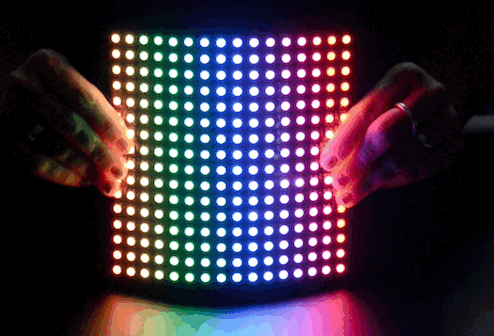# NeoPixl

neopixel模块可使用Neopixel（WS2812）（单独可寻址的RGB LED条带）与Microbit连接。注意使用该neopixel模块时，需要单独导入：

``import neopixel``NeoPixels是多色可编程LED。

#### 类

``clear()``

``show()``

#### 操作

``````np = (255,0,128)#第一元件
np[-1] = (0,255,0)#最后一个元素
np.show()#仅显示更新的值``````

``print(np)``

#### 使用Neopixels

``````import neopixel
np = neopixel.NeoPixel(pin0, 8)``````

``np = (255, 0, 0)``

``np[-1] = (255, 0, 255)``

``print(np)``

``np.show()``

#### 例子：

``````"""
neopixel_random.py

Repeatedly displays random colours onto the LED strip.
This example requires a strip of 8 Neopixels (WS2812) connected to pin0.

"""
from microbit import *
import neopixel
from random import randint

# Setup the Neopixel strip on pin0 with a length of 8 pixels
np = neopixel.NeoPixel(pin0, 8)

while True:
#Iterate over each LED in the strip

for pixel_id in range(0, len(np)):
red = randint(0, 60)
green = randint(0, 60)
blue = randint(0, 60)

# Assign the current LED a random red, green and blue value between 0 and 60
np[pixel_id] = (red, green, blue)

# Display the current pixel data on the Neopixel strip
np.show()
sleep(100)``````